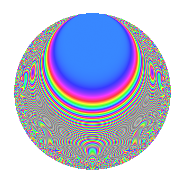# Properties

 Label 287.2.jLevel 287 Weight 2 Character orbit j Rep. character $$\chi_{287}(81,\cdot)$$ Character field $$\Q(\zeta_{6})$$ Dimension 52 Newforms 1 Sturm bound 56 Trace bound 0

# Related objects

## Defining parameters

 Level: $$N$$ = $$287 = 7 \cdot 41$$ Weight: $$k$$ = $$2$$ Character orbit: $$[\chi]$$ = 287.j (of order $$6$$ and degree $$2$$) Character conductor: $$\operatorname{cond}(\chi)$$ = $$287$$ Character field: $$\Q(\zeta_{6})$$ Newforms: $$1$$ Sturm bound: $$56$$ Trace bound: $$0$$

## Dimensions

The following table gives the dimensions of various subspaces of $$M_{2}(287, [\chi])$$.

Total New Old
Modular forms 60 60 0
Cusp forms 52 52 0
Eisenstein series 8 8 0

## Trace form

 $$52q - 2q^{2} - 26q^{4} - 6q^{5} - 12q^{8} + 16q^{9} + O(q^{10})$$ $$52q - 2q^{2} - 26q^{4} - 6q^{5} - 12q^{8} + 16q^{9} + 16q^{10} - 26q^{16} + 12q^{18} + 16q^{20} - 48q^{21} - 32q^{25} + 6q^{31} + 20q^{32} - 20q^{33} - 4q^{36} - 24q^{37} + 14q^{39} + 44q^{40} + 24q^{41} - 2q^{42} - 32q^{43} + 26q^{45} + 12q^{46} + 16q^{49} - 12q^{51} + 8q^{57} + 2q^{59} + 14q^{61} - 20q^{62} - 20q^{64} - 4q^{66} - 42q^{72} - 16q^{73} - 22q^{74} - 32q^{77} + 88q^{78} - 52q^{80} - 46q^{81} - 42q^{82} - 112q^{83} + 72q^{84} + 16q^{86} - 18q^{87} + 224q^{90} + 86q^{91} - 32q^{92} - 48q^{98} + O(q^{100})$$

## Decomposition of $$S_{2}^{\mathrm{new}}(287, [\chi])$$ into irreducible Hecke orbits

Label Dim. $$A$$ Field CM Traces $q$-expansion
$$a_2$$ $$a_3$$ $$a_5$$ $$a_7$$
287.2.j.a $$52$$ $$2.292$$ None $$-2$$ $$0$$ $$-6$$ $$0$$